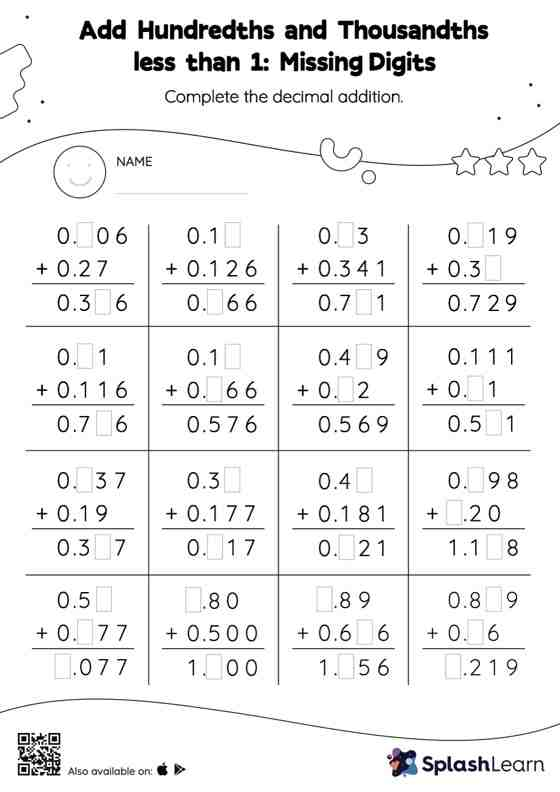# Add Hundredths and Thousandths less than 1: Missing Digits Worksheet

Home > Add Hundredths and Thousandths less than 1: Missing DigitsChildren use their math skills to complete this add hundredths and thousandths less than 1 worksheet. When adding decimals on this worksheet, students align the decimal points and use zero as a placeholder. Then to find the missing number in the add hundredths and thousandths less than 1 worksheet, students then apply the relationship between addition and subtraction. In each problem, the numbers are laid out in the vertical format. In this format, pair of digits in each successive place are tackled one by one. This helps students follow a structured approach.